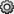Documentation

## Save and Load Parts of Variables in MAT-Files

You can save and load parts of variables directly in MAT-files without loading them into memory using the `matfile` function. The primary advantage of using the `matfile` function over the `load` or `save` functions is that you can process parts of very large data sets that are otherwise too large to fit in memory. When working with these large variables, read and write as much data into memory as possible at a time. Otherwise, repeated file access can negatively impact the performance of your code.

### Save and Load Using the `matfile` Function

This example shows how to load, modify, and save part of a variable in an existing MAT-file using the `matfile` function.

Create a Version 7.3 MAT-file with two variables, `A` and `B`.

```A = rand(5); B = magic(10); save example.mat A B -v7.3; clear A B```

Construct a `MatFile` object from the MAT-file, `example.mat`. The `matfile` function creates a `MatFile` object that corresponds to the MAT-file and contains the properties of the `MatFile` object. By default, `matfile` only permits loading from existing MAT-files.

`exampleObject = matfile('example.mat');`

To enable saving, call `matfile` with the `Writable` parameter.

`exampleObject = matfile('example.mat','Writable',true);`

Alternatively, construct the object and set `Properties.Writable` in separate steps.

```exampleObject = matfile('example.mat'); exampleObject.Properties.Writable = true;```

Load the first row of `B` from `example.mat` into variable `firstRowB` and modify the data. When you index into objects associated with Version 7.3 MAT-files, MATLAB® loads only the part of the variable that you specify.

```firstRowB = exampleObject.B(1,:); firstRowB = 2 * firstRowB;```

Update the values in the first row of variable `B` in `example.mat` using the values stored in `firstRowB`.

`exampleObject.B(1,:) = firstRowB;`

For very large files, the best practice is to read and write as much data into memory as possible at a time. Otherwise, repeated file access negatively impacts the performance of your code. For example, suppose that your file contains many rows and columns, and that loading a single row requires most of the available memory. Rather than updating one element at a time, update each row.

```[nrowsB,ncolsB] = size(exampleObject,'B'); for row = 1:nrowsB exampleObject.B(row,:) = row * exampleObject.B(row,:); end```

If memory is not a concern, you can update the entire contents of a variable at a time.

`exampleObject.B = 10 * exampleObject.B;`

Alternatively, update a variable by calling the `save` function with the `-append` option. The `-append` option requests that the `save` function replace only the specified variable, `B`, and leave other variables in the file intact. This method always requires that you load and save the entire variable.

```load('example.mat','B'); B(1,:) = 2 * B(1,:); save('example.mat','-append','B');```

Add a variable to the file using the `matlab.io.MatFile` object.

`exampleObject.C = magic(8);`

You also can add the variable by calling the `save` function with the `-append` option.

```C = magic(8); save('example.mat','-append','C'); clear C```

### Load Parts of Variables Dynamically

This example shows how to access parts of variables from a MAT-file dynamically. This is useful when working with MAT-files whose variables names are not always known.

Consider the example MAT-file, `topography.mat`, that contains one or more arrays with unknown names. Construct a `MatFile` object that corresponds to the file, `topography.mat`. Call `who` to get the variable names in the file.

```exampleObject = matfile('topography.mat'); varlist = who(exampleObject)```
```varlist = 4x1 cell array {'topo' } {'topolegend'} {'topomap1' } {'topomap2' } ```

`varlist` is a cell array containing the names of the four variables in `topography.mat`.

The third and fourth variables, `topomap1` and `topomap2`, are both arrays containing topography data. Load the elevation data from the third column of each variable into a field of the structure array, `S`. For each field, specify a field name that is the original variable name prefixed by `elevationOf_`. Then, access the data in each variable as properties of `exampleObject`. Because `varName` is a variable, enclose it in parentheses.

```for index = 3:4 varName = varlist{index}; S(1).(['elevationOf_',varName]) = exampleObject.(varName)(:,3); end```

View the contents of the structure array, `S`.

`S`
```S = struct with fields: elevationOf_topomap1: [64x1 double] elevationOf_topomap2: [128x1 double] ```

`S` has two fields, `elevationOf_topomap1` and `elevationOf_topomap2`, each containing a column vector.

### Avoid Inadvertently Loading Entire Variables

When you do not know the size of a large variable in a MAT-file and want to load only parts of that variable at a time, avoid using the `end` keyword. Using the `end` keyword temporarily loads the entire contents of the variable in question into memory. For very large variables, loading takes a long time or generates `Out` `of` `Memory` errors. Instead, call the `size` method for `MatFile` objects.

For example, this code temporarily loads the entire contents of `B` in memory:

`lastColB = exampleObject.B(:,end);`

Use this code instead to improve performance:

```[nrows,ncols] = size(exampleObject,'B'); lastColB = exampleObject.B(:,ncols);```

Similarly, any time you refer to a variable with syntax of the form `matObj.varName`, such as `exampleObject.B`, MATLAB® temporarily loads the entire variable into memory. Therefore, make sure to call the `size` method for `MatFile` objects with syntax such as:

`[nrows,ncols] = size(exampleObject,'B');`

rather than passing the entire contents of `exampleObject.B` to the `size` function,

`[nrows,ncols] = size(exampleObject.B);`

The difference in syntax is subtle, but significant.

### Partial Loading and Saving Requires Version 7.3 MAT-Files

Any load or save operation that uses a `MatFile` object associated with a Version 7 or earlier MAT-file temporarily loads the entire variable into memory.

Use the `matfile` function to create files in Version 7.3 format. For example, this code

```newfile = matfile('newfile.mat'); ```

creates a MAT-file that supports partial loading and saving.

However, by default, the `save` function creates Version 7 MAT-files. Convert existing MAT-files to Version 7.3 by calling the `save` function with the `-v7.3` option, such as:

```load('durer.mat'); save('mycopy_durer.mat','-v7.3');```

To change your preferences to save new files in Version 7.3 format, access the Environment section on the Home tab, and click. Select MATLAB > General > MAT-Files. This preference is not available in MATLAB Online™.

Download ebook